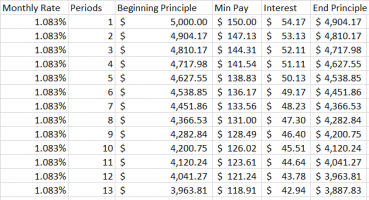Calculating Number of Payments if there is a variable payment amount

Jebediahstewart34

New member
I understand there are very simple formulas to calculate the nper if you have a defined interest rate, PV, FV and PMT. I'm running into an issue however in a scenario with a variable payment amount (e.g. the payment is either 3% of the balance or $25, whichever is greater). I know I can build out an amortization schedule and simply count the number of periods until FV ends up hitting 0, but is there a formula I can use to get the same result? I'll continue trying to a spreadsheet with the amort table momentarily, having issues getting it to attach. EDIT: I adjusted the payment scenario noted above to ensure the balance would reduce with each min pay. It also appears I'm unable to attach a spreadsheet. Not sure if that's user error or not, but it's a simple spreadsheet with columns of Monthly Rate, Period, Beginning Principle, Min Pay, Interest and End Principle. It simply calculates each of those fields for each subsequent period until the End Principle gets to$0, which then shows how many periods it took to get there.

Last edited:

JeffM

Elite Member
$\text p = \text {amount of loan.}$
$n = \text {number of payments at 3 percent per month.}$
$\dfrac{log_{10}(25/p)}{log_{10}(1 - 0.03)} \text { is an integer} \implies n =\dfrac{log_{10}(25/p)}{log_{10}(1 - 0.03)}.$.

$\dfrac{log_{10}(25/p)}{log_{10}(1 - 0.03)} \text { not an integer} \implies n =\left \lceil \dfrac{log_{10}(25/p)}{log_{10}(1 - 0.03)} \right \rceil.$.

Obviously the number of payments will be 1 more than n.

Jebediahstewart34

New member
Thanks for the reply. I'm not getting the same result using that formula compared to the full amortization table.
Full Scenario:
Principle amount of loan = $5,000 APR = 13.0% therefore monthly rate = 1.083% Payment = Max(25, .03*ending_balance_current_period) Using the formula noted above, it shows n=(-2.30103/-0.01322827) which equals 173.95 Using the full amort table, the min pay stays at 3% of the balance until period 94, after which it stays at 25 for the remainder of the loan. With the total number of payments ending up at 135. Here's a screen shot for the first few periods to give you an idea of what I have so far. Any ideas as to why they may be different?JeffM Elite Member You did not say anything about an explicit interest rate. You merely said that the payment was 3% per month. You did not say 3% of what. How is “balance“ described contractually? Let’s start over with you providing complete information. Jebediahstewart34 New member Apologies for any confusion. The situation is a financial example where a person is responsible for repaying a debt by making a minimum payment until the balance is completely paid off. Initial Balance:$5,000
Ending Balance: $0 Monthly Interest Rate: 1.083% Variable Min Payment: Either$25, or 3% of the remaining outstanding balance from the prior period. Whichever of those two amounts is greater.

Attempting to solve for the total number of payments. Primary issue being that most formulas I've seen are simple calculations if the payment amount is fixed. I have not found a way to account for the variable payment where the actual $amount changes every period until 3% of the prior period's balance is no longer greater than$25.

JeffM

Elite Member
Apologies for any confusion. The situation is a financial example where a person is responsible for repaying a debt by making a minimum payment until the balance is completely paid off.

Initial Balance: $5,000 Ending Balance:$0
Monthly Interest Rate: 1.083%

JeffM

Elite Member
I think your formula from post #2 needs slight modifications:
1. To account for the interest one would use (1-0.03 + 0.13/12) instead of (1-0.03);
2. The target principal value should be $25/0.03, not$25, and thus (25/p) needs to be replaced by (25/(0.03*p)). With this changes I get the value of 92.5843 months, whose ceiling is 93 months.
@blamocur

Looks good to me.

Of course, in practice, we would have to know how grace days work (if there are any), whether interest is credited daily or monthly. To get an exact formula, you must have the contractual language.